# NCERT solution for Class 6 Maths Chapter 14: Practical Geometry

In this page we have NCERT solution for Class 6 Maths Chapter 14: Practical Geometry . Hope you like them and do not forget to like , social share and comment at the end of the page.

## Chapter 14 Exercise 14.1

Question 1:
Draw a circle of radius 3.2 cm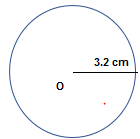Step 1 Open the compasses for the required radius of 3.2 cm.
Step 2 Mark a point with a sharp pencil where we want the centre of the circle to
be. Name it as O.
Step 3 Place the pointer of the compasses on O.
Step 4 Turn the compasses slowly to draw the circle.

Question 2:
With the same centre O, draw two circles of radii 4 cm and 2.5 cm.
Step 1 Open the compasses for the required radius of 2.5 cm.
Step 2 Mark a point with a sharp pencil where we want the centre of the circle to be. Name it as O.
Step 3 Place the pointer of the compasses on O.
Step 4 Turn the compasses slowly to draw the circle.
Step 5. Now Open the compasses for the required radius of 4 cm.
Step 6. Place the pointer of the compasses on O and Turn the compasses slowly to draw the circle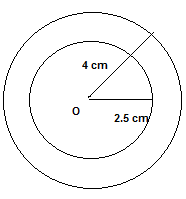Question 3:
Draw a circle and any two of its diameters. If you join the ends of these diameters, what is the figure obtained? What figure is obtained if the diameters are perpendicular to each other? How do you check your answer?
(i) By joining the ends of two diameters, we get a rectangle. By measuring, we find AD = BC, AC = BD i.e., pairs of opposite sides are equal and∠A =∠B=∠C=∠D= 90 i.e. each angle is of 90°. Hence, it is a rectangle.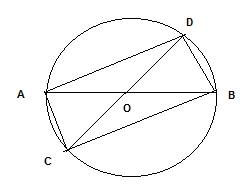(ii) If the diameters are perpendicular to each other, then by joining the ends of two diameters, we get a square. By measuring, we find that AB = BC = CD = DA, i.e., all four sides are equal. Also∠A=∠B=∠C= L∠D= 90′, i.e. each angle is of 90′. Hence, it is a square.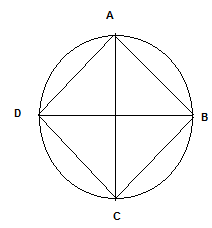Question 4:
Draw any circle and mark points A, B and C such that
(a) A is on the circle.
(b) B is in the interior of the circle.
(c) C is in the exterior of the circle.
(1) Point O is marked by using the pencil and then circle’s centre is drawn
(2) Compasses pointer is placed at O after that circle is drawn slowly using the compasses
Now points are marked as below
A is on the circle.
B is in the interior of the circle.
C is in the exterior of the circle.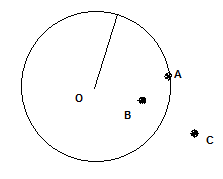Question 5:
Let A, B be the centers of two circles of equal radii; draw them so that each one
of them passes through the centre of the other. Let them intersect at C and D.
Examine whether AB and CD are at right angles
Draw two circles of equal radii taking A and B as their centre such that one of them passes through the centre of the other. They intersect at C and D. Join AB and CD. Yes, AB and CD intersect at right angle as∠COB is 90.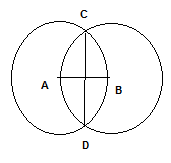## Chapter 14 Exercise 14.2

Question 1:
Draw a line segment of length 7.3 cm using a ruler.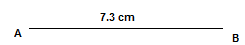(i)At the point A make place the zero mark of the ruler
(ii)And pick appoint B at the exact distance of 7.3 cm from the point A
(iii) Now join AB

Question 2:
Construct a line segment of length 5.6 cm using ruler and compasses.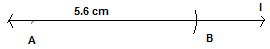(i) Draw a line l. Mark a point A on a line l.
(ii) Place the compasses pointer on the zero mark of the ruler. Open it to place the pencil point up-to the 5.6 cm mark.
(iii) Now place the pointer of compasses on A and swing an arc to cut l at B.

Question 3:
Construct AB of length 7.8 cm. From this, cut off AC of length 4.7 cm. Measure BC.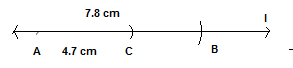(i) Draw a line l. Mark a point A on a line l.
(ii) Place the compasses pointer on the zero mark of the ruler. Open it to place the pencil point up-to the 7.8 cm mark.
(ii) Now place the pointer of compasses on A and swing an arc to cut l at B.
(iii) Now Place the compasses pointer on the zero mark of the ruler. Open it to place the pencil point up-to the 4.7 cm mark.
(iv) Now place the pointer of compasses on A and swing an arc to cut l at C.
(v) Now when you measure the length BC with the help of ruler, you will find it as 3.1 cm

Question 4:
Given AB of length 3.9 cm, construct PQ such that the length of PQ is twice that of AB. Verify by measurement.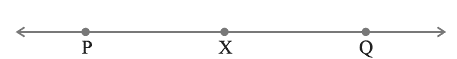(Hint: Construct PX such that length of PX = length of AB; then cut off XQ such that XQ also has the length of AB.)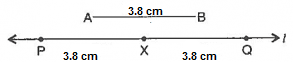(i) A Line is drawn ‘l’ and a point P is taken on it
(ii) Draw line segment AB of length 3.9 cm
(iii) Now by adjusting the compass to the length AB and placing the pointer of compasses on P and swing an arc to cut l at X.
(iv) Now placing the pointer of compasses on X and swing an arc to cut l at Q.
Now, measurement can find that PQ=7.8 cm
=2 X 3.9 cm

Question 5:
Given AB of length 7.3 cm and CD of length 3.4 cm, construct a line segment XY such that the length of XY is equal to the difference between the lengths of AB and CD. Verify by measurement
(i) First draw AB of length 7.3 cm and CD of length 3.4 cm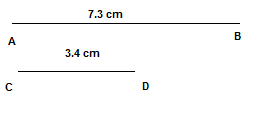(ii) Now by adjusting the compass to the length CD and placing the pointer of compasses on A and swing an arc to cut l at P.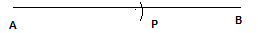(iii) Now Draw Line is drawn ‘l’ and a point X is taken on it
(iv) Now by adjusting the compass to the length PB and placing the pointer of compasses on A and swing an arc to cut l at Y.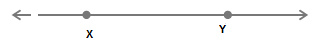XY=7.3-3.4=3.9 cm

## Chapter 14 Exercise 14.3

Question 1:
Draw any line segment PQ. Without measuring PQ, construct a copy of PQ.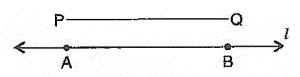(i) First, we draw a line segment PQ
(ii) Now construct a line of length ‘l’. Point A is marked
(iii) Now by adjusting the compass to the length PQ and placing the pointer of compasses on A and swing an arc to cut l at B.
NowAB is the copy ofPQ

Question 2.
Given some line segment AB, whose length you do not know, construct PQ such that the length of PQ is twice that of AB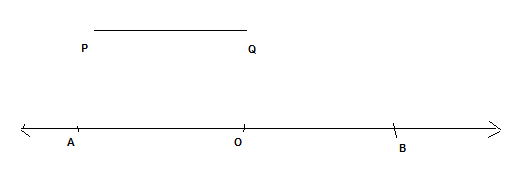(i) Draw the line segment PQwhose certain length is unknown
(ii) Now construct a line of length ‘l’. Point A is marked
(iii) Now by adjusting the compass to the length PQ and placing the pointer of compasses on A and swing an arc to cut l at O.
(iv) Now again keeping the same length on the compass and placing the pointer of compasses on O and swing an arc to cut l at B.
(v)Now AB =2PQ

## Chapter 14 Exercise 14.4

Question 1:
Draw any line segment AB. Mark any point M on it. Through M, draw a perpendicular to AB.
(use ruler and compasses)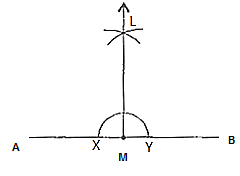(i) Draw the line segment AB
(ii) Make a point M on it
(iii) With M as midpoint and with some radius, an intersecting arc is drawn the line at 2 points X and Y.
(iv) With X and Y as centers as well as radius more than OX, draw 2 arcs, they cut together at L.
(v) Join LM Then LM is perpendicular to PQ through O point.

Question 2:
Draw any line segment PQ. Take any point R not on it. Through R, draw a perpendicular to PQ. (use ruler and set-square)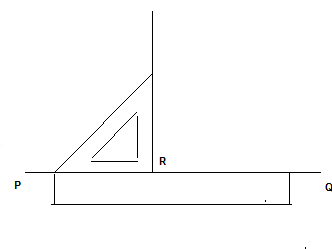(i)A set-square is placed onPQsuch that one arm of its right angle aligns along PQ
(ii) A ruler is placed along the edge opposite to the right angle of the set-square.
(iii) Hold the ruler fixed. Set square slide the along the ruler till the point R touches set square’s other arm
(iv) Hold the set-square firmly in this position. Draw RT along the edge of the set-square.

Question 3:
Draw a line l and a point X on it. Through X, draw a line segment XY perpendicular to l.
Now draw a perpendicular to XY at Y. (use ruler and compasses)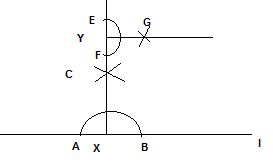(i)Make a line ‘l’ and take point X on it.
(ii) With X as centre and a certain radius, an arc is draw at intersecting the line ‘l’ at 2 points A and B.
(iii) With P and Q as centers and a radius greater than XA, draw 2 arcs, which cut each other at C.
(iv) Join XC and produce it to Y. Then XY is perpendicular to ‘l’
(v) With Y as centre and a certain radius, draw an arc intersecting XYat two points E and F.
(vi) With E and F as centers and radius greater than YE, draw 2 arcs and they cut each other at G.
(vii) Join YG, then YG is perpendicular to XY at Y

## Exercise 14.5

Question 1:
Draw AB of length 7.3 cm and find its axis of symmetry.
Axis of symmetry of line segmentAB will be the perpendicular bisector ofAB. So, we need to draw the perpendicular bisector of AB.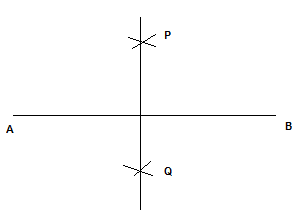(i)Draw a line segment is drawn AB = 7.3 cm
(ii) WithA and B as centers and radius more than half of AB, construct 2 arcs which intersect each other at P andQ.
(iii) Join PQ. Then PQ is the symmetry axis of the segment line AB.

Question 2:
Draw a line segment of length 9.5 cm and construct its perpendicular bisector.
(i)Draw a line segment is drawn AB = 9.5 cm
(ii) WithA and B as centers and radius more than half of AB, construct 2 arcs which intersect each other at P andQ.
(iii) Join PQ. Then PQ is the symmetry axis of the segment line AB.Question 3:
Draw the perpendicular bisector of XY whose length is 10.3 cm.
(a) Take any point P on the bisector drawn. Examine whether PX = PY.
(b) If M is the mid-point of XY, what can you say about the lengths MX and XY?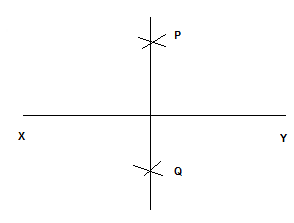(i)Draw a line segment is drawnXY= 10.3 cm
(ii) With X and Y as centre and radius more than half of XY, draw two arcs which intersect each other at A and B.
(iii) Join AB. Then AB is the required perpendicular bisector ofXY

Now,
(iv) Point P is taken on the drawn bisector. By using the divider and we can check that thatPX=PB
(v) If M is the midpoint ofXY, thenMX=MY=1/2(XY)

Question 4:
Draw a line segment of length 12.8 cm. Using compasses, divide it into four equal parts. Verify by actual measurement.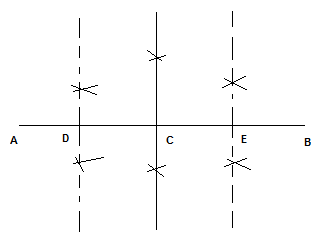(i)Draw a line segment is drawn AB = 12.8 cm
(ii)A perpendicular bisector of line AB is drawn which cuts it at a point C. So, is the mid-point of AB
(iii) A perpendicular bisector of line AC is drawn which cuts it at D. So, D is the midpoint of AC
(iv) Again, A perpendicular bisector of line CB is drawn which cuts it at E. So, E is the midpoint of CB
v) Thus, point D, C, E divides the line segmentAB in the 4 equal parts.
(6) By actual measurement, we can find thatAD=DC=CE=EB=4.2 cm

Question 5:
With PQ of length 6.1 cm as diameter, draw a circle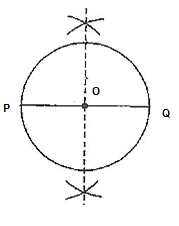(i)Draw a line segment PQ = 6.1 cm.
(ii)A perpendicular bisector is drawn which cuts, it at O. So, O is the mid-point of PQ.
(iii) WithO as centre and OP as s radius, draw a circle. Now PQ will be the diameter of the circle drawn

Question 6:
Draw a circle with centre C and radius 3.4 cm. Draw any chord AB. Construct the perpendicular bisector of AB and examine if it passes through C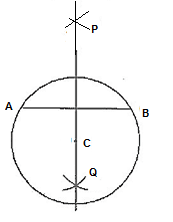(i) With centre C draw a circle and radius 3.4 cm.
(ii)Draw a ChordAB
(iii) With A and B as centers and radius more than half of AB, make 2 arcs which cut each other at P and Q.
(iv) Join PQ. So, PQ is the perpendicular bisector ofAB
(v) You will notice, it will pass through the center C of the circle

Question 7:
Repeat Question 6, if AB happens to be a diameter.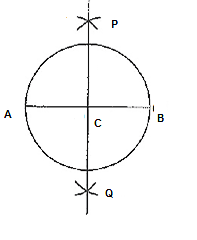(i)Draw a circle with centre C and radius 3.4 cm.
(ii)AB diameter is drawn which passes through the point C
(iii) With A and B is taken as centers and radius more than half of it, make 2 arcs which intersect each other at P and Q.
(iv) Join XY. Then XY is the perpendicular bisector ofAB
(v) You will notice, it will pass through the center C of the circle

Question 8:
Draw a circle of radius 4 cm. Draw any two of its chords. Construct the perpendicular bisectors of these chords. Where do they meet?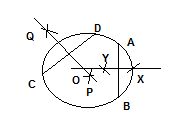(i) Draw the circle with O and radius 4 cm.
(ii) Draw any two chords AB and CD in this circle.
(iii) Taking A and B as centers and radius more than half AB, draw two arcs which intersect each other at points X and Y
(iv) Join XY. Then XY is the perpendicular bisector of chord AB.
(v) Similarly draw PQ the perpendicular bisector of chord CD.
(vi) We will notice that These two perpendicular bisectors meet at O, the centre of the circle.

Question 9:
Draw any angle with vertex O. Take a point A on one of its arms and B on another such that OA = OB. Draw the perpendicular bisectors of OA and OB.
Let them meet at P. Is PA = PB?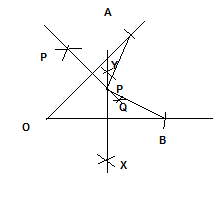(I)Draw any angle with vertex O.
(ii) Take a point A on one of its arms and B on another such that OA = OB.
(iii) Draw perpendicular bisector of OA as PQ and perpendicular bisector of OB as XY
(iv) Let them meet at P. Join PA and PB.
(v) With the help of divider, we check that PA =PB.

## Chapter 14 Exercise 14.6

Question 1:
Draw ∠POQ of measure 75° and find its line of symmetry.
(a) Construct a line l and mark a point O on it.
(b) Place the pointer of the compasses at O and draw an arc of any radius which intersects the line l at A.
(c) Taking same radius, with centre A, cut the previous arc at B.
(d) Join OB, then∠BOA=60°
(e) Taking same radius, with centre B, cut the previous arc at C.
(f) Draw bisector of∠BOC. The angle is of∠BOC. Mark, it at D. Thus,∠DOA=90°
(g) DrawOP as bisector of∠DOB
Thus,∠POA=75°
(h) DrawOX as bisector of∠POA
Thus,∠XOA=37.5°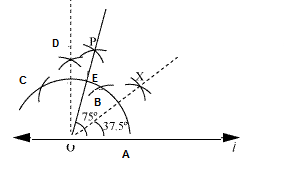Question 2:
Draw an angle of measure 147° and construct its bisector
(a) Draw a lineOA
(b) Using protractor, construct∠AOB=147°
(c) Taking the centre as O and any suitable radius, draw an arc which cuts the armsOAandOB at X and Y respectively.
(d) Taking X as the centre and radius more than half of XY, draw an arc.
(e) Taking Y as centre and with the same radius, draw another arc which cut the previous at Z.
(f) Join OZ and produce it.
(g) Thus,OZ is the required bisector of∠AOB.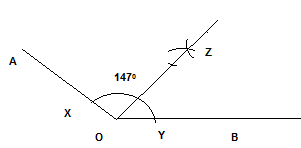Question 3:
Draw a right angle and construct its bisector
(a) Draw a line PQ and take a point O on it.
(b) Taking O as centre and convenient radius, draw an arc which intersects PQ at A and B.
(c) Taking A and B as centers and radius more than half of AB, draw two arcs which intersect each other at X.
(d) Join OX. Thus,∠XOQis the required right angle.
(e) Taking B and C as centre and radius more than half of BC, draw two arcs which intersect each other at the point Y.
(f) Join OY. Thus,OYis the required bisector of∠XOQ.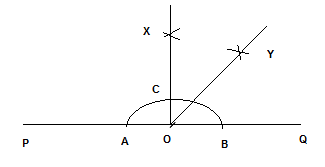Question 4:
Draw an angle of measure 153° and divide it into four equal parts.
(a) Draw a rayOP
(b) At O, with the help of a protractor, construct∠QOP=1530
(c) DrawOS as the bisector of∠QOP.
(d) Again, drawOT as bisector of∠QOS
(e) Again, drawOR as bisector of∠SOP.
(f) Thus,OT,OS andOR divide∠AOBin four equal parts.Question 5:
Construct with ruler and compasses, angles of following measures:
(a) 60° (b) 30° (c) 90° (d) 120° (e) 45° (f) 135°
(1)60°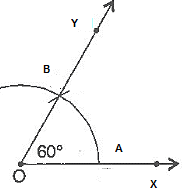(i) Draw a rayOX
(ii) Taking O as centre and convenient radius, mark an arc, which intersectsOXat A.
(iii) Taking A as centre and same radius, cut previous arc at B.
(iv) Join OB.
The,∠YOX is required angle of 600

(2)30°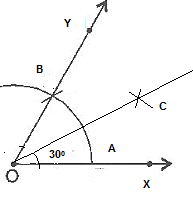(i) Draw the angle 60o as given previous question
(ii) Put the pointer on A and mark an arc.
(iii) Put the pointer on B and with same radius, cut the previous arc at C.
(iv) Join OC
Thus,∠COX is required angle of 300

(3)90°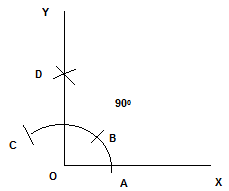(i) Draw a rayOX
(ii) Taking O as centre and convenient radius, mark an arc, which intersectsA at O X.
(iii) Taking A as centre and same radius, cut previous arc at B.
(iv) Taking B as centre and same radius, draw another arc intersecting the same arc at C.
(v) Taking C and B as centers and same radius, draw two arcs intersecting each other at D.
(vi) Join OD and produce it to form a ray OY
The,∠YOX is required angle of 900

(4)120°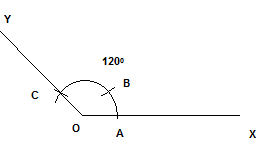(i) Draw a rayOX
(ii) Taking O as centre and convenient radius, mark an arc, which intersectsOX at A.
(iii) With A as centre and same radius, cut previous arc at B.
(iv) Now with B as centre and same radius cut the arc at C.
(v) Join OC.
The,∠YOX is required angle of 1200

(5)45°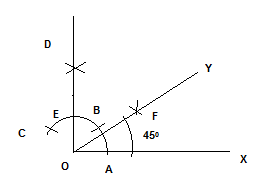(i) Draw a rayOX
(ii) Taking O as centre and convenient radius, mark an arc, which intersectsOA at X.
(iii) With A as centre and same radius, cut previous arc at B.
(iv) With B as centre and same radius, draw another arc intersecting the same arc at E.
(v) With B and C as centers and same radius, draw two arcs intersecting each other at D.
(vi) Join OD. The,∠DOA is required angle of 900
(vii) Now Draw the bisector of ∠DOA. With E and A as center, draw two arcs intersecting each other at F and Join OF
Thus,∠YOX is required angle of 450

(6)135°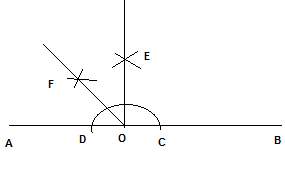(i) Draw a line AB and take a point O on it.
(ii) Taking O as centre and convenient radius, mark an arc, which intersects AB at C and D
(iii) With C and D as centers and radius more than half of CD, draw two arcs intersecting each other at E.
(iv) Join OE. Thus,∠AOE =90°.
(v) DrawOFthe bisector of∠AOE.
The,∠BOF is required angle of 135°

Question 6:
Draw an angle of measure 45°and bisect it.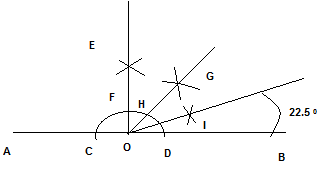(i) Draw a line AB and take a point O on it.
(ii) With O as centre and a convenient radius, draw an arc which intersects AB at two points C and D
(iii) With C and D as centers and radius more than half of AC, draw two arcs which intersect each other at E.
(iv)Join OE. Then∠EOB is an angle of 90
(v) DrawOG as the bisector of∠EOB. Thus,∠GOB =45°
(vi) Again, drawOIas the bisector of∠GOB.
Thus,∠IOB =22.5 °

Question 7:
Draw an angle of measure 135° and bisect it.
(i) Draw a line AB and take a point O on it.
(ii) Taking O as centre and convenient radius, mark an arc, which intersects AB at C and D
(iii) With C and D as centers and radius more than half of CD, draw two arcs intersecting each other at E.
(iv) Join OE. Thus,∠AOE =90°.
(v) DrawOFthe bisector of∠AOE.
The,∠BOF is required angle of 135°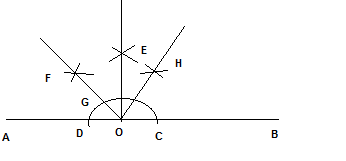(vi) With G and C as centers and radius more than half of GC, draw two arcs intersecting each other at H
(vii) Join OH
The,∠HOB =67.5°

Question 8:
Draw an angle of 70° Make a copy of it using only a straight edge and compasses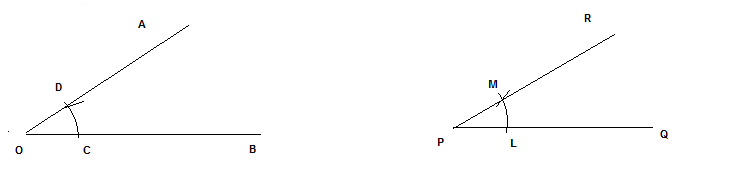(i) Draw an angle 70° with protractor, ∠AOB =70°
(ii) Draw the line PQ
(iii)Place the compasses at O and draw an arc to cut the rays of∠AOB at C and D.
(iv) Use the same compasses, setting to draw an arc with P as centre, cutting PQ at L.
(v) Set your compasses setting to the length CD with the same radius.
(vi) Place the compasses pointer at L and draw the arc to cut the arc drawn earlier at M.
(vii) Join PM and extend the line
So∠RPQ =70°

Question 9:
Draw an angle of 400 Copy its supplementary angle.
(i) Draw an angle of 40° with the help of protractor, naming∠AOB.
(ii) Draw a line PQ and Take any point R on PQ.
(iii)Place the compasses at O and draw an arc to cut the rays of∠AOB at C and D.
(iv) Use the same compasses, setting to draw an arc with R as centre, cutting PQ at L.
(v) Set your compasses setting to the length CD with the same radius.
(vi) Place the compasses pointer at L and draw the arc to cut the arc drawn earlier at M.
(vii) Join RM and extend the line
(viii)So,∠SRQ =40° and∠PRS is supplementary of it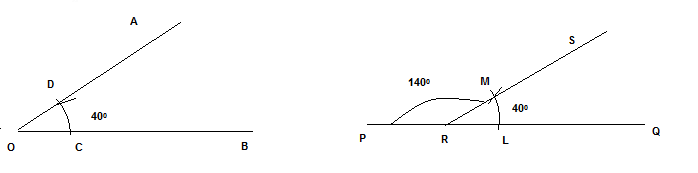• Ncert Solutions### Practice Question

Question 1 What is $\frac {1}{2} + \frac {3}{4}$ ?
A)$\frac {5}{4}$
B)$\frac {1}{4}$
C)$1$
D)$\frac {4}{5}$
Question 2 Pinhole camera produces an ?
A)An erect and small image
B)an Inverted and small image
C)An inverted and enlarged image
D)None of the above